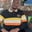Related Tags

python
python3
communitycreator

# How to use a lambda function to solve a problem in PythonWale Moshood

### Overview

A Python lambda function is a function that has one expression but can take any number of arguments. It is a small anonymous function that is subject to a more restrictive but concise syntax than regular Python functions.

### Syntax

lambda arguments : expression

### Example

a = lambda x, y : x * y

print(a(5, 6))

The example above multiplies arguments x and y and returns the result, 30.

### How to use lambda

#### Anonymous functions

An anonymous function is a function without a name. In Python, an anonymous function is created with the lambda keyword, which may or may not be assigned a name.

For example, consider a two-argument anonymous function that is defined with lambda but is not bound to a variable. The lambda is not given a name. The function below defines a lambda expression, takes two arguments, and returns their sum.

lambda a, b : a + b

#### Single expression

A Python lambda function is a single-line expression in contrast to other regular functions. Although you can spread the expression over multiple lines using parentheses or multi-line strings, it still remains a single-line expression.

The example below returns the even string when the lambda argument is even, and odd when the argument is odd.

(lambda x:
(x % 2 and 'even' or 'odd'))

### Arguments

The lambda function supports all the different ways of passing arguments, such as a normal function object defined with the def keyword.

This includes:

• #### Positional arguments

>>> (lambda a, b, c: a + b + c) (1, 2, 3)
6
• #### Variable list of arguments

>>> (lambda *args: sum(args))(1,2,3)
6
• #### Variable list of keyword arguments

>>> (lambda **kwargs: sum(kwargs.values()))(one=1, two=2, three=3)
6

• #### Keyword-only arguments

>>> (lambda x, *, y=0, z=0: x + y + z)(1, y=2, z=3)
6

### Single function definition

lambda expression allows the same function definition to make two or more functions in the same program.

### Example

def myfunc(x):
return lambda a : a * x

mydoubler = myfunc(3)
mytripler = myfunc(4)

print(mydoubler(11))
print(mytripler(11))

### Conclusion

This shot summarizes Python lambda functions, using lambda as an anonymous function, and writing lambda as a normal Python function.

RELATED TAGS

python
python3
communitycreator

CONTRIBUTORWale Moshood
RELATED COURSES

View all Courses

Keep Exploring

Learn in-demand tech skills in half the time